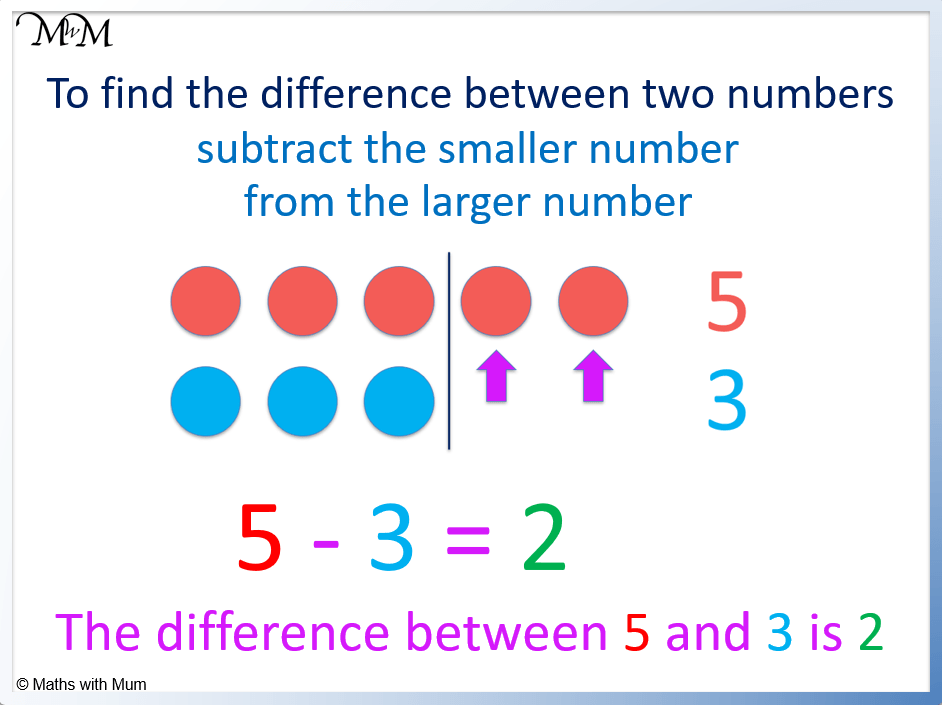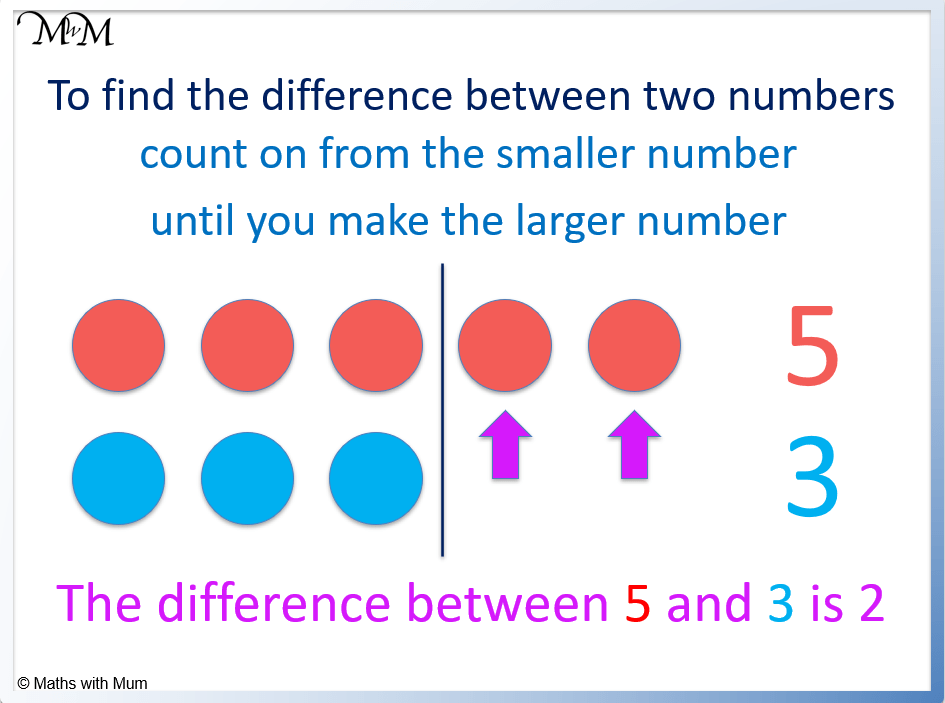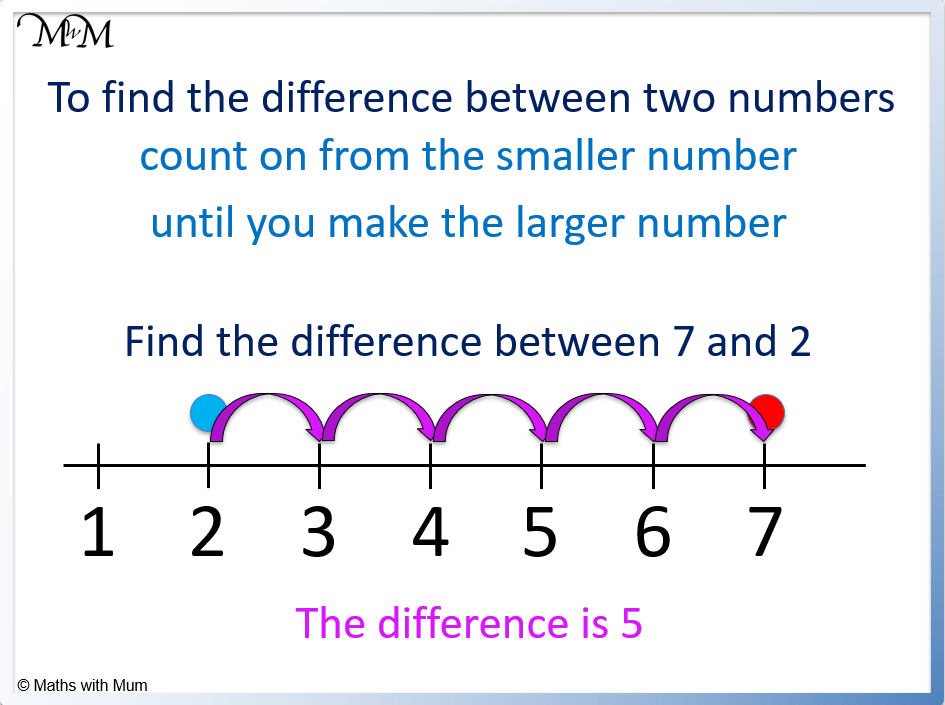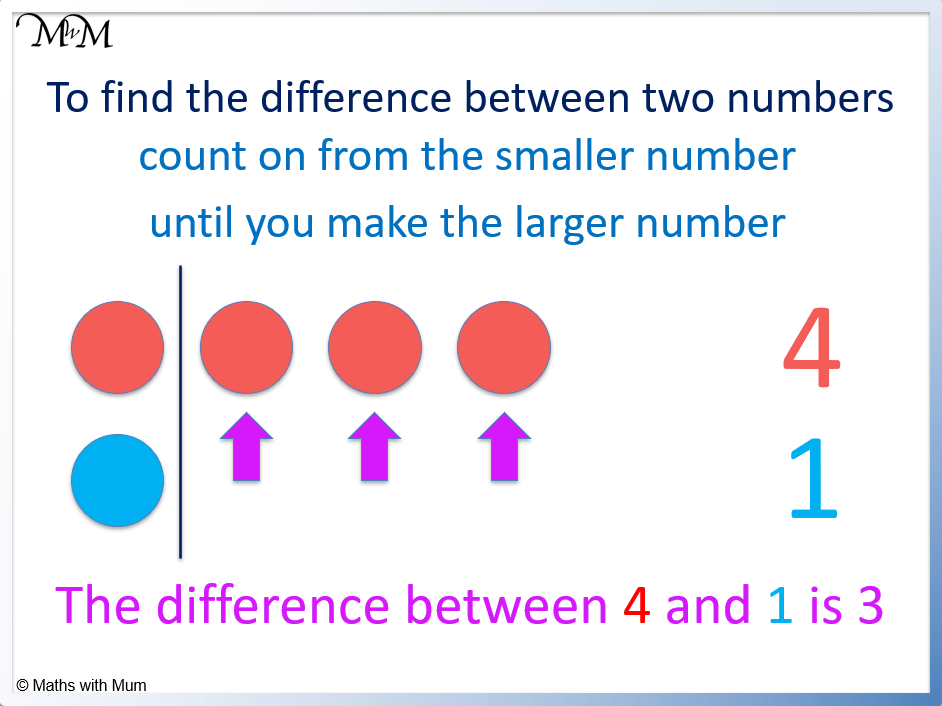# How to Find the Difference Between Two Numbers

How to Find the Difference Between Two NumbersTo find the difference between two numbers, subtract the smaller number from the larger number.• To find the difference between two numbers, subtract the smaller number from the larger number.
• 5 – 3 = 2 and so, the difference between 5 and 3 is 2.
• Alternatively, the difference between two numbers can be found by counting on from the smaller number.
• Count on from the smaller number until you make the larger number. This amount is the difference.• The difference between 7 and 2 is 5 because 7 – 2 = 5.
• Number lines can be used to find the difference between two numbers.
• Start at the smaller number and count on in jumps until you reach the larger number.
• There are 5 jumps from 2 to 7 and so, the difference between 2 and 7 is 5.Supporting Lessons

# The Difference Between Numbers

## How to Find the Difference Between Two Numbers

To find the difference between two numbers, subtract the smaller number from the larger number. For example, the difference between 5 and 3 is 2 because 5 – 3 = 2.No matter how large the numbers are, subtracting the smaller number from the larger number will always calculate the difference.

For example, find the difference between 80 and 20.

The larger number is 80 and the smaller number is 20.

Therefore to calculate the difference between the numbers, subtract 20 from 80.

80 – 20 = 60 and so, the difference between 80 and 20 is 60.## Teaching How to Find the Difference Between Two Numbers

To teach finding the difference:

1. Use physical objects such as counters to help count on
2. Use number lines to show the size of numbers
3. Show that subtraction gives us the difference

When introducing the idea of a difference between numbers, it is best to start with very small numbers that can be represented with physical objects, such as counters.

Placing the counters side by side can be used to show the difference in size between one number and another.

For example, in the image below we can see that 4 contains 3 more counters than 1 and so, the difference between 4 and 1 must be 3.After the idea of what the difference between two numbers means is understood, it is time to increase the size of the numbers and look at numbers on a number line.

Number lines are useful tools to use for teaching the difference between two numbers. Mark both numbers on the number line and count the number of jumps needed to move from the smaller number to the larger number.

For example, the difference between 6 and 3 can be seen to be 3 because there are 3 jumps needed to move from the smaller number to the larger number.## How to Find the Difference Between Two Numbers Formula

The formula for the difference between two numbers, a and b is a-b. Where a is the largest number and b is the smallest number.For example, the difference between 25 and 13.## The Difference Between Two-Digit Numbers

To find the difference between two-digit numbers, subtract the smaller number from the larger. With two-digit numbers, the subtraction is most easily calculated using vertical column subtraction.

For example, calculate the difference between 56 and 33.

Line up the digits and subtract vertically.

In the ones column, 6 – 3 = 3.

In the tens column, 5 – 3 = 2.56 – 33 = 23.

## How to Find the Difference Between Two Odd Numbers

The difference between two odd numbers is always even. For example, 25 – 13 – 12, which is even.All odd numbers have a difference which is even. This is because consecutive odd numbers always differ by 2. Adding 2 to an odd number takes us to the next odd number.

## How to Find the Difference Between Two Even Numbers

The difference between two even numbers is always even. For example, 16 – 12 = 4, which is even.All even numbers have a difference which is even. This is because even numbers are all multiples of 2. Every even number is in the two times table. To get from one even number to the next, add two.

## How to Find the Difference Between Two Negative Numbers

The difference between two negative numbers is the same as the difference between these numbers with positive signs. For example the difference between -8 and -1 is 7 because the difference between 8 and 1 is 7.Now try our lesson on Subtraction Number Sentences where we learn how to write subtraction number sentences.error: Content is protected !!